## RD Sharma Class 10 Solutions Chapter 3 Pair of Linear Equations in Two Variables Ex 3.11

These Solutions are part of RD Sharma Class 10 Solutions. Here we have given RD Sharma Class 10 Solutions Chapter 3 Pair of Linear Equations in Two Variables Ex 3.11

Other Exercises

Question 1.
If in a rectangle, the length is increased and breadth reduced each by 2 units, the area is reduced by 28 square units. If, however the length is reduced by 1 unit and the breadth increased by 2 units, the area increased by 33 square units. Find the area of the rectangle.
Solution:
Let the length of rectangle = x units
Area = Length x breadth = x x y = xy sq. units
According to the condition given,
(x + 2) (y – 2) = xy – 28
=> xy – 2x + 2y – 4 = xy – 28
=> -2x + 2y = -28 + 4 = -24
=> x – y = 12 ….(i)
(Dividing by -2)
and (x – 1) (y + 2) = xy + 33
=> xy + 2x – y – 2 = xy + 33
=> 2x – y = 33 + 2
=> 2x – y = 35 ….(ii)
Subtracting (i), from (ii)
x = 23
Substituting the value of x in (i)
23 – y = 12
=> -y = 12 – 23 = -11
=> y = 11
Area of the rectangle = xy = 23 x 11 = 253 sq. units

Question 2.
The area of a rectangle remains the same if the length is increased by 7 metres and the breadth is decreased by 3 metres. The area remains unaffected if the length is decreased by 7 metres and breadth is increased by 5 metres. Find the dimensions of the rectangle.
Solution:
Let the length of a rectangle = x m
Area = Length x breadth = xy sq. m
According to the condition given,
(x + 7)(y – 3) = xy
=> xy – 3x + 7y – 21 = xy
-3x + 7y = 21 ….(i)
and (x – 7) (y + 5) = xy
=>xy + 5x – 7y = xy
5x – 7y = 35 …(ii)
(i) from (ii)
2x = 56
=> x = 28
Substituting the value of x in (i)
-3 x 28 + 7y = 21
– 84 +7y = 21
=> 7y = 21 + 84 = 105
y = 15
Length of the rectangle = 28 m and breadth = 15 m

Question 3.
In a rectangle, if the length is increased by 3 metres and breadth is decreased by 4 metres, the area of the rectangle is reduced by 67 square metres. If length reduced by 1 metre and breath increased by 4 metres, the area is increased by is 89 sq. metres. Find the dimensions of the rectangle.
Solution:
Let length of rectangle = x m
Area = Length x breadth = xy m2
According to the given condition,
(x + 3) (y – 4) = xy – 67
=> xy – 4x + 3y – 12 = xy – 67
=>-4x + 3y = -67+ 12 = -55
=> 4x – 3y = 55 ….(i)
and (x -1) (y + 4) = xy + 89
=> xy + 4x – y – 4 = xy + 89
=> 4x – y = 89 + 4 = 93 ….(ii)
Subtracting (i) from (ii)
2y = 38
=> y = 19
Substituting the value of y in (i)
4x – 3 x 19 = 55
=> 4x – 57 = 55
=> 4x = 55 + 57 = 112
=> x = 28
Length of the rectangle = 28 m and breadth = 19 m

Question 4.
The incomes of X and Y are in the ratio of 8 : 7 and their expenditures are in the ratio 19 : 16. If each saves ₹ 1250, find their incomes.
Solution:
Incomes of X and Y are in the ratio 8 : 7
and their expenditures = 19 : 16
Let income of X = ₹ 8x
and income of Y = ₹ 7x
and let expenditures of X = 19y
and expenditure of Y = 16y
Saving in each case is save i.e. ₹ 1250
8x – 19y = 1250 ….(i)
and 7x – 16y = 1250 ….(ii)
Multiplying (i) by 7 and (ii) by 8, we get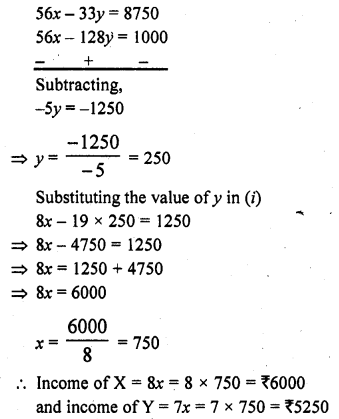Question 5.
A and B each has some money. If A gives ₹ 30 to B, then B will have twice the money left with A, but if B gives ₹ 10 to A, then A will have thrice as much as is left with B. How much money does each have?
Solution:
Let A’s money = 7 x
and B’s money = 7 y
According to the given conditions,
2(x – 30) = 7 + 30
=> 2x – 60 = y + 30
=> 2x – y = 30 + 60 = 90 ….(i)
and x + 10 = 3 (y – 10)
=> x + 10 = 3y – 30
=> x – 3y = -30 – 10 = -40 ………(ii)
Multiplying (i) by 3 and (ii) by 1,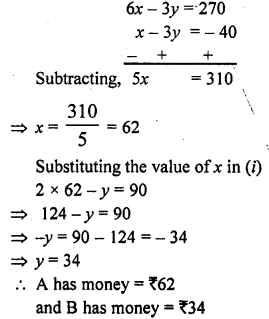Question 6.
ABCD is a cyclic quadrilateral such that ∠A = (4y + 20)°, ∠B = (3y – 5)°, ∠C = (+4x)° and ∠D = (7x + 5)°. Find the four angles. [NCERT]
Solution: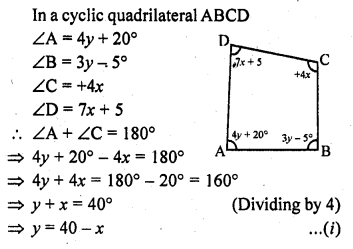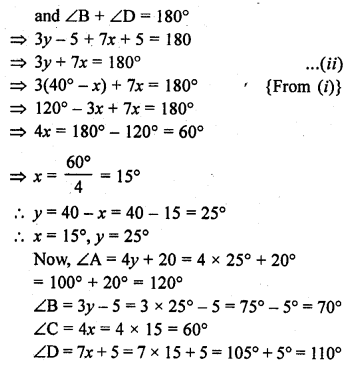Question 7.
2 men and 7 boys can do a piece of work in 4 days. The same work is done in 3 days by 4 men and 4 boys. How long would it take one man and one boy to do it ?
Solution:
Let one man can do a work is = x days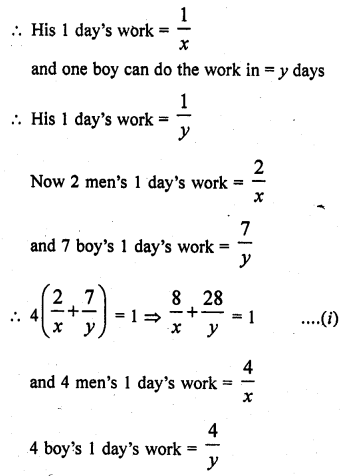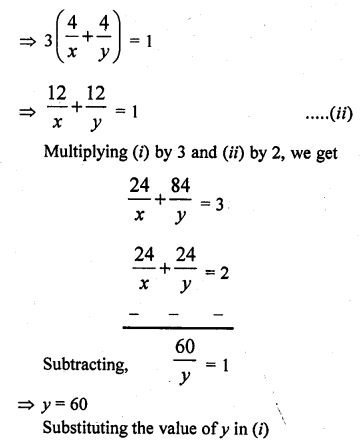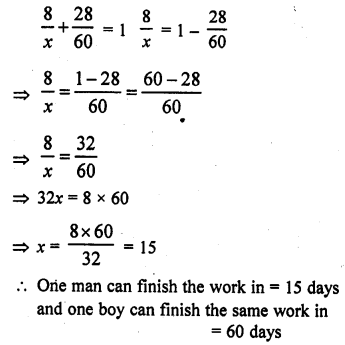Question 8.
In a ∆ABC, ∠A = x°, ∠B = (3x – 2)°, ∠C = y°. Also ∠C – ∠B = 9°. Find the three angles.
Solution:
We know that sum of three angles of a triangle = 180°
∠A + ∠B + ∠C = 180°
=> x° + (3x – 2)° + y° = 180°
=> x + 3x – 2 + y = 180
=> 4x + y = 180 + 2 = 182 …(i)
∠C – ∠B = 9
y – (3x – 2) = 9
=> y – 3x + 2 = 9
=> y – 3x = 9 – 2
=> -3x + y = 7 ….(ii)
Subtracting (ii) from (i)
7x = 175 => x = 25
Substituting the value of x in (ii)
-3 x 25 + y = 7
=> -75 + y = 7
=> y = 7 + 75 = 82
∠A = x° = 25°
∠B = 3x – 2 = 3 x 25 – 2 = 73°
∠C = 7 = 82°

Question 9.
In a cyclic quadrilateral ABCD, ∠A = (2x + 4)°, ∠B = (y + 3)°, ∠C = (2y + 10)°, ∠D = (4x – 5)°. Find the four angles.
Solution:
and ∠A = (2x + 4)°, ∠B = (y + 3)°, ∠C = (2y + 10)°, ∠D = (4x – 5)°
The sum of opposite angles = 180°
∠A + ∠C=180° and ∠B + ∠D = 180°
=> 2x + 4 + 2y + 10 = 180°
=> 2x + 2y + 14 = 180°
=> 2x + 2y = 180 – 14 = 166
=> x + y = 83 ….(i)
(Dividing by 2)
and y + 3 + 4x – 5 = 180°
=> 4x + 7 – 2 = 180°
4x + 7 = 180°+ 2= 182° ….(ii)
Subtracting (i) from (ii)
3x = 99
=> x = 33
Substituting the value of x in (i)
33 + y = 83
=> y = 83 – 33 = 50
∠A = 2x + 4 = 2 x 33 + 4 = 66 + 4 = 70°
∠B = y + 3 = 50 + 3 = 53°
∠C = 2y + 10 = 2 x 50 + 10 = 100 + 10 = 110°
∠D = 4x – 5 = 4 x 33 – 5 = 132 – 5 = 127°

Question 10.
Yash scored 40 marks in a test, getting 3 marks for each right answer and losing 1 mark for each wrong answer. Had 4 marks been awarded for each correct answer and 2 marks been deducted for each incorrect answer, then Yash would have scored 50 marks. How many questions were there in the test ?
Solution:
Let number of right answers questions = x
and number of wrong answers questions = y
Now according to the given conditions,
3x – y = 40 ….(i)
and 4x – 2y = 50
=> 2x – y = 25 ….(ii)
(Dividing by 2)
Subtracting (ii) from (i), x = 15
Substituting the value of x in (i)
3 x 15 – y = 40
=> 45 – y = 40
=> y = 45 – 40 = 5
x = 15 and y = 5
Total number of questions = 15 + 5 = 20

Question 11.
In a ∆ABC, ∠A = x°, ∠B = 3x° and ∠C = 7°. If 3y – 5x = 30, prove that the triangle is right angled.
Solution:
In a ∆ABC ,
∠A = x°, ∠B = 3x° and ∠C = 7°
But ∠A + ∠B + ∠C = 180° (Sum of angles of a triangle)
=> x + 3x + y = 180
=>4x + y = 180 ………(i)
and 3y – 5x = 30 ….(ii)
from (i) y = 180 – 4x
Substituting the value of y in (ii)
3 (180 – 4x) – 5x = 30
540 – 12x – 5x = 30
=> -17x = -540 + 30 = -510
=> 17x = 510
=> x = 30
y = 180 – 4x = 180 – 4 x 30 = 180 – 120 = 60
∠A = x = 30°
∠B = 3x = 3 x 30° = 90°
∠C = y = 60°
∠B of ∆ABC = 90°
∆ABC is a right angled triangle.

Question 12.
The car hire charges in a city comprise of a fixed charges together with the charge for the distance covered. For a journey of 12 km, the charge paid is ₹ 89 and for a journey of 20 km, the charge paid is ₹ 145. What will a person have to pay for travelling a distance of 30 km?
Solution:
Let the fixed charges = ₹ x
and charges per km = ₹ y
According to the given conditionsQuestion 13.
A part of monthly hostel charges in a college are fixed and the remaining depend on the number of days one has taken food in the mess. When a student A takes food for 20 days, he has to pay ₹ 1000 as hostel charges whereas a student B, who takes food for 26 days, pays ₹ 1180 as hostel charges. Find the fixed charged and the cost of food per day. [CBSE 2000]
Solution:
Let the fixed charge of the college hostel = ₹ x
and daily charges = ₹ y
According to the given conditions,
x + 20y = 1000 …(i)
x + 26y =1180 …(ii)
Subtracting (i) from (ii), we get
6y = 180
=> y = 30
Substituting the value of y in (i)
x + 20 x 30 = 1000
=> x + 600 = 1000
=> x = 1000 – 600 = 400
Fixed charges = ₹ 400 and daily charges = ₹ 30

Question 14.
Half the perimeter of a garden whose length is 4 m more them its width is 36 m. Find the dimensions of the garden.
Solution:
Let the length of the garden = x m
and width = y m
x – y = 4 …(i)
and x + y = 36 ….(ii)
2x = 40
=> x = 20
and subtracting,
2y = 32
y = 16
Length of the garden = 20 m and width = 16 m

Question 15.
The larger of two supplementary angles exceeds the smaller by 18 degrees. Find them
Solution:
In two supplementary angles,
Let larger angle = x
and smaller angle = y
x – y = 18°
But x + y = 180°
2x = 198°
=> x = 9°
Subtracting the get,
2y = 162°
=> y = 81°
Angles are 99° and 81°

Question 16.
2 women and 5 men can together finish a piece of embroidery in 4 days while 3 women and 6 men can finish it in 3 days. Find the time taken by 1 woman alone to finish the embroidery and that taken by 1 man alone.
Solution:
Let one woman can do the work in = x days
and one man can do the same work = y days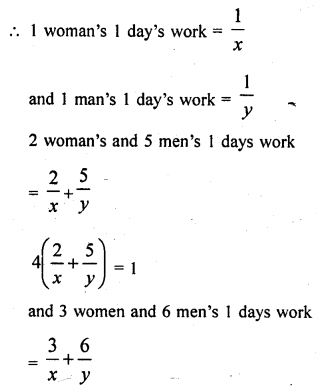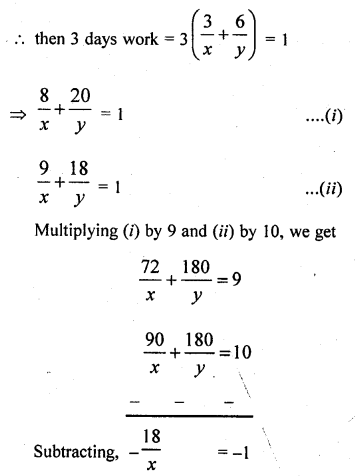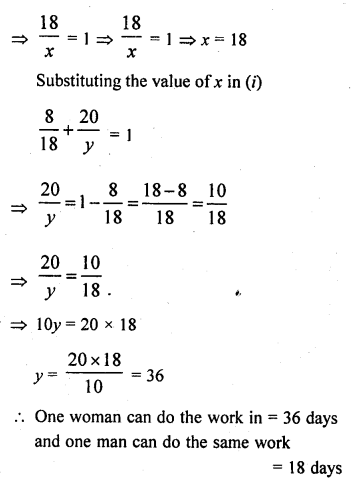Question 17.
Meena went to a bank to withdraw ₹ 2000. She asked the cashier to give her ₹ 50 and ₹ 100 notes only. Meena got 25 notes in all. Find how many notes ₹ 50 and ₹ 100 she received.
Solution:
Total amount with drawn = ₹ 2000
Let number of ₹ 50 notes = x
and of ₹ 100 = y
According to the conditions
x + y = 25 …(i)
and 50x + 100y = 2000
=> x + 2y = 40 ….(ii)
(Dividing by 50)
Subtracting (i) from (ii), y = 15
Substituting the value of y in (i)
x + y = 25
x + 15 = 25
=> x = 25 – 15 = 10
Number of 50 rupees notes = 10
and number of 100 rupee notes = 15

Question 18.
There are two examination rooms A and B. If 10 candidates are sent from A to B, the number of students in each room is same. If 20 candidates are sent from B to A, the number of students in A is double the number of students in B. Find the number of students in each room.
Solution:
Let A examination room has students = x
and B room has students = y
According to the given conditions,
x – 10 = y + 10
=> x – y = 10 + 10
=> x – y = 20 ….(i)
and x + 20 = 2 (y – 20)
=> x + 20 = 2y – 40
=> x – 2y = – 40 – 20 = – 60 ….(ii)
Subtracting (ii) from (i), y = 80
Substituting the value of y in (i)
x – 80 = 20
=> x = 20 + 80 = 100
In examination room A, the students are = 100
and in B room = 80

Question 19.
A railway half ticket costs half the full fare and the reservation charge is the same on half ticket as on full ticket. One reserved first class ticket from Mumbai to Ahmedabad costs ₹ 216 and one full and one half reserved first class tickets cost ₹ 327. What is the basic first class full fare and what is the reservation charge?
Solution:
Let the rate of fare of full ticket = ₹ x
and rate of reservation = ₹ y
According to the given conditions,
x + y = 216 …(i)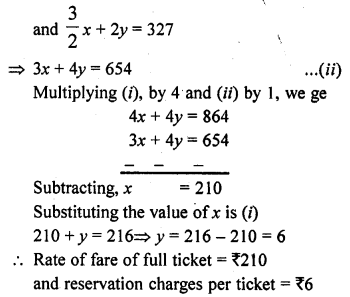Question 20.
A wizard having powers of mystic in candations and magical medicines seeing a cock, fight going on, spoke privately to both the owners of cocks. To one he said; if your bird wins, than you give me your stake-money, but if you do not win, I shall give you two third of that. Going to the other, he promised in the same way to give three fourths. From both of them his gain would be only 12 gold coins. Find the stake of money each of the cock- owners have.
Solution:
Let the first owner of cock has state money = x gold coins
and second owner has = y gold coins
According to the given conditions,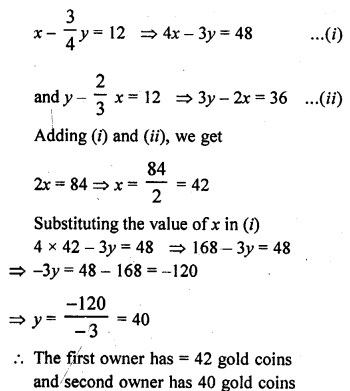Question 21.
The students of a class are made to stand in rows. If 3 student are extra in a row, there would be 1 row less. If 3 students are less in a row there would be 2 rows more. Find the number of students in the class.
Solution:
Let number of rows = x
and number of students in each row = y
Now according to the conditions given
Number of total students = xy
(y + 3)(x – 1) = xy
=> xy – y + 3x – 3 = xy
=>3x – y = 3 …(i)
and (y – 3) (x + 2) = xy
=> xy + 2y – 3x – 6 = xy
=> 2y – 3x = 6 …(ii)
Adding (i) and (ii), y = 9
Substituting the value of y in (i)
3x – 9 = 3
=> 3x = 3 + 9 = 12
=> x = 4
Number of total students = xy = 4 x 9 = 36

Question 22.
One says, “give me hundred, friend ! I shall then become twice as rich as you”. The other replies, “If you give me ten, I shall be six times as rich as you”. Tell me what is the amount of their respective capital ?
Solution:
Let first person has amount of money = Rs. x
and second person has = Rs. y
Now according to the conditions given,
x + 100 = 2(y – 100)
=> x + 100 = 2y – 200
=> x – 2y = – 200 – 100
=> x – 2y = -300 ………(i)
and 6(x – 10) = (y + 10)
=> 6x – 60 = y + 10
=> 6x – y = 10 + 60
=> 6x – y = 70 ……….(ii)
From (i), x = 2y – 300
Substituting the value of x in (ii)
6(2y – 300) – y = 70
=> 12y – 1800 – y = 70
=> 11y = 70 + 1800 = 1870
y = 170
x = 2y – 300 = 2 x 170 -300 = 340 – 300 = 40
First person has money = ₹ 40
and second person has = ₹ 170

Question 23.
A shopkeeper sells a saree at 8% profit and a sweater at 10% discount, thereby getting a sum of ₹ 1008. If she had sold the saree at 10% profit and the sweater at 8% discount, she would have got ₹ 1028. Find the cost price of the saree and the list price (price before discount) of the sweater. [NCERT Exemplar]
Solution:
Let the cost price of the saree and the list price of the sweater be ₹ x and ₹ y, respectively.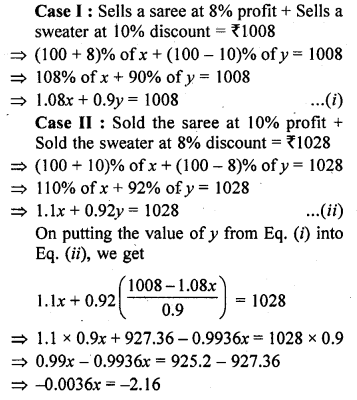Question 24.
In a competitive examination, one mark is aw awarded for each correct answer while $$\frac { 1 }{ 2 }$$ mark is deducted for every wrong answer. Jayanti answered 120 questions and got 90 marks. How many questions did she answer correctly. [NCERT Exemplar]
Solution:
Let x be the number of correct answer of the questions in a competitive examination,
then (120 – x) be the number of wrong answers of the questions.
Then, by given condition,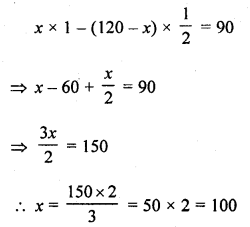Hence, Jayanti answered correctly 100 questions.

Question 25.
A shopkeeper gives books pn rent for reading. She takes a fixed charge for the first two days, and an additional charge for each day thereafter. Latika paid ₹ 22 for a book kept for 6 days, while Anand paid ₹ 16 for the book kept for four days. Find the fixed charges and charge for each extra day. [NCERT Exemplar]
Solution:
Let Latika takes a fixed charge for the first two day is ₹ x and additional charge for each day thereafter is ₹ y.
Now, by first condition,
Latika paid ₹ 22 for a book kept for six days
i.e., x + 4y = 22 …(i)
and by second condition,
Anand paid ₹ 16 for a book kept for four days
i.e., x + 2y = 16 …(ii)
Now, subtracting Eq. (ii) from Eq. (i), we get
2y = 6 => y = 3
On putting the value of y in Eq. (ii), we get
x + 2 x 3 = 16
x = 16 – 6 = 10
Hence, the fixed charge = ₹ 10
and the charge for each extra day = ₹ 3

Hope given RD Sharma Class 10 Solutions Chapter 3 Pair of Linear Equations in Two Variables Ex 3.11 are helpful to complete your math homework.

If you have any doubts, please comment below. Learn Insta try to provide online math tutoring for you.